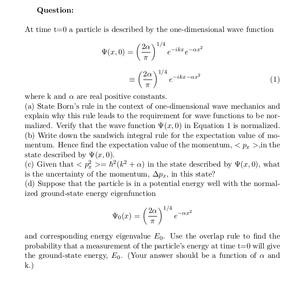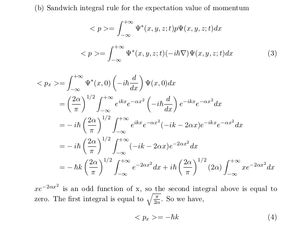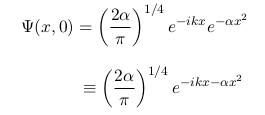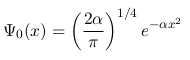Solved Problems in Physics, Physical Chemistry, and Computer Simulation Projects for College and Graduate Students.# One-Dimensional Wave Function: Born’s Rule, Sandwich Integral and Overlap Rules

Regular price
\$3.00
Sale price
\$3.00
Tax included.

Question:
At time t=0 a particle is described by the one-dimensional wave functionwhere k and α are real positive constants.
(a) State Born’s rule in the context of one-dimensional wave mechanics and explain why this rule leads to the requirement for wave functions to be normalized. Verify that the wave function Ψ(x, 0) in Equation 1 is normalized.

(b) Write down the sandwich integral rule for the expectation value of momentum. Hence find the expectation value of the momentum, < px >,in the state described by Ψ(x, 0).

(c) Given thatin the state described by Ψ(x, 0), what is the uncertainty of the momentum, ∆px , in this state?

(d) Suppose that the particle is in a potential energy well with the normalized ground-state energy eigenfunctionand corresponding energy eigenvalue E0 . Use the overlap rule to find the probability that a measurement of the particle’s energy at time t=0 will give the ground-state energy, E0 . (Your answer should be a function of α and k.)

By purchasing this product, you will get the step by step solution of the above problem in pdf format and the corresponding latex file where you can edit the solution. I am also available to help  you with any possible question you may have. If you have any question about this solution, please send it to info@solutions4students.com

I'm available to help students with their homework assignments and computer simulation projects.